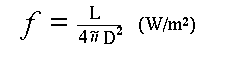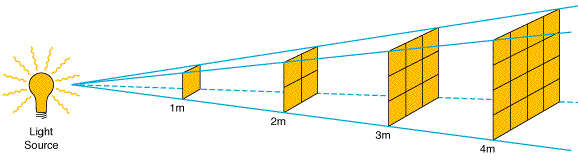## Magnitude Scales

Astronomy is an ancient science. As such, it carries with it some very archaic conventions. The magnitude system, invented in 150 B.C., is one of them.The BRIGHTEST stars, were called FIRST magnitude. Stars that appear half as bright were SECOND magnitude, those dimmer still were called THIRD, etc. The LARGER the number, the DIMMER the star. Objects BRIGHTER than the brightest star (1st magnitude), are expressed as negative values. There are two kinds of magnitudes:

APPARENT MAGNITUDE, small m, refers to the flux of objects based on what we see on Earth, and will be affected by the distance of the source.

ABSOLUTE MAGNITUDE, capitol M. This is a measure of an intrinsic property of the star or object, and is thus independent of distance. There is a decided 'standard distance', 10 parsecs, from which all stars can be fairly compared. A stars absolute magnitude is simply its apparent magnitude if placed at 10 parsecs. Only a few stars are this near to us, so nearly all stars have an absolute magnitude that is brighter than their apparent magnitude.

### The Inverse Square law

 The Flux we recieve from an object and the Luminosity (Intrinsic Brightness) of that object is related by the distance in this way: Once you know the distance to the star, D, you can determine its intrinsic luminosity, L, based on how bright it is (the flux, F,) on earth and the inverse square law.Notice in the illustration above, if you double the distance away, the area shadowed increases by a factor of FOUR. This is the same as saying you have one fourth the flux (energy per square inch) at twice the distance. It's all because of that inverse (Distance)2 term!